International
Tables for
Crystallography
Volume H
Powder diffraction
Edited by C. J. Gilmore, J. A. Kaduk and H. Schenk

International Tables for Crystallography (2018). Vol. H, ch. 1.1, pp. 11-15

Section 1.1.4. The peak profile

R. E. Dinnebiera* and S. J. L. Billingeb,c

aMax-Planck-Institute for Solid State Research, Heisenbergstrasse 1, D-70569 Stuttgart, Germany,bDepartment of Applied Physics and Applied Mathematics, Columbia University, 500 West 120th Street, Room 200 Mudd, MC 4701, New York, NY 10027, USA, and cCondensed Matter Physics and Materials Science Department, Brookhaven National Laboratory, PO Box 5000, Upton, NY 11973–5000, USA
Correspondence e-mail:  r.dinnebier@fkf.mpg.de

1.1.4. The peak profile

| top | pdf |

The peak profile refers to the shape of the measured Bragg peak. In the treatment above, the Bragg peaks from a perfect infinite crystal were delta functions and therefore infinitely narrow. In reality, the finite size of the crystal, the finite resolution of the measurement and defects in the material that result in inhomogeneous strains all broaden the delta function, giving it a finite width and some characteristic shape. When fitting a model to the measured diffraction pattern we should correctly account for these effects in order to obtain correct values for the Bragg-peak intensities. On the other hand, a careful study of the peak shapes yields important information about the size of the crystallites in the sample and defects that they contain. With recent improvements in instrumentation and computational data-analysis methods, this latter type of study has become more important and is having considerable scientific and technological impact.

The convolution theorem of the Fourier transform that was introduced in the derivation of the crystallographic structure factor above is also very useful in understanding the peak profile. In this case, the measured Bragg peak can be thought of as a delta function convoluted with a profile (Klug & Alexander, 1974). The profile of the Bragg reflection hkl,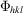, can be written as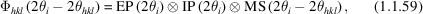where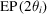is the emission profile of the X-ray source (tube or synchrotron),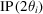contains additional contributions to the profile from the instrument and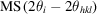is the contribution from the microstructure of the sample. The symbol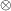denotes convolution.

The convolution of two functions f(t) and g(t) in real space is defined as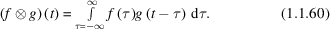The convolution theorem tells us that the Fourier transform (FT) of two convoluted functions is the product of the Fourier transforms of those functions: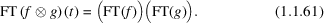Normalization of the transform leads to scaling factors like 2π which have been omitted here for simplicity.

In practice, numerical integrations are almost always required, as many of the instrument aberration functions cannot be convoluted analytically. This convolution approach is the basis of the so-called fundamental-parameter (FP) approach (Cheary & Coelho, 1992) and has proven to be superior to other more empirical or phenomenological methods. The idea behind the FP approach is to build up the profile from first principles, exclusively using measurable physical quantities like slit widths, slit lengths, Soller-slit opening angles etc. The process of convolution from a fundamental-parameters perspective is an approximation whereby second- and higher-order effects are typically neglected for computational speed and simplicity. The instrumental profile is usually fully characterized by measuring a line-profile standard such as NIST SRM 660c LaB6, which is expected to contain only small microstructural contributions, and comparing the calculated diffraction pattern to the measured one. Once the instrumental part of the profile is sufficiently well determined, it can be assumed that the remaining contributions to the `real' profile are purely sample dependent (e.g. domain size, strain).

In general, it is desirable to keep the number of functions that are used to describe the peak profile to a minimum. Typical examples of mathematical functions which are convoluted to form the profile of a Bragg reflection include:

(a) the hat function H (e.g. for all kinds of rectangular slits),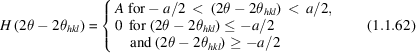(Fig. 1.1.18a);Figure 1.1.18 | top | pdf |Normalized peak-shape functions. (a) The hat function, (b) the Gaussian function and (c) the Lorentzian function.

(b) the normalized Gaussian G (e.g. for microstrain broadening),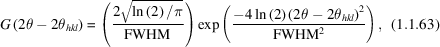(Fig. 1.1.18b), where FWHM denotes the full width at half maximum of the Gaussian function in ° 2θ; and

(c) the Lorentzian function L (e.g. for the emission profile),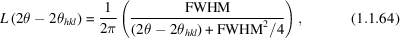(Fig. 1.1.18c).

These functions can be convoluted sequentially as needed, first with the delta-function Bragg peak, and subsequently with the existing profile from the previous convolutions, each time resulting in a new profile that can become quite complex (Fig. 1.1.19). It is often the case that for a particular resolution effect the angular dependence of the profile function is known from the geometry of the measurement, and the convolution function for each peak is determined with only a very small number of parameters.Figure 1.1.19 | top | pdf |Peak fits of three selected reflections for an LaB6 standard measured with Mo Kα1 radiation (λ = 0.7093 Å) from a Ge(220) monochromator in Debye–Scherrer geometry using the fundamental-parameter approach. (a) A pure Lorentzian emission profile with a half width of 0.2695 mÅ is applied, refining the peak position and intensity only; (b) additionally, a hat shape function of the receiving slit in the equatorial plane with a width of 0.1 mm has been convoluted into the profile; (c) additionally, an axial convolution with filament-, sample- and receiving-slit lengths of 8 mm each and a secondary Soller slit with an opening angle of 2.5° has been convoluted into the profile; (d) additionally a small contribution of Gaussian broadening coming from the position-sensitive detector is convoluted into the profile. [From Mittemeijer & Welzel (2012). Copyright Wiley-VCH Verlag GmbH & Co. KGaA. Reproduced with permission.]

1.1.4.1. Sample contributions to the peak profile

| top | pdf |

Features of the sample that affect the peak profile include crystallite domain size and shape, dislocations, disclinations, twin and stacking faults, antiphase domains, microstrains, grain surface relaxations, and compositional fluctuations. Here we reproduce some basic results as examples; they also illustrate some fundamental aspects of diffraction from real crystals.

1.1.4.1.1. Crystallite size

| top | pdf |

The starting point for the analysis of finite size effects is the Laue equation, equation (1.1.39), which is reproduced here for a one-dimensional crystal: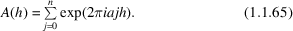When we were deriving the Bragg equation from the Laue equation we assumed an infinite crystal, and the sum taken to infinity resulted in delta functions at the reciprocal-lattice points. Now we want to consider a finite crystal with n unit cells. There is an analytic form for this sum which, using Euler's identity, is given by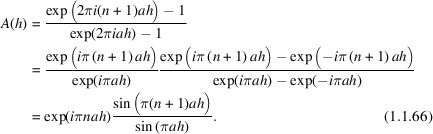The intensity is obtained by taking the modulus squared of this complex function, resulting in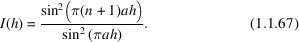This function has sharp maxima when h = ν(1/a), where ν is an integer. This large central maximum falls off with a width proportional to 1/n2 with oscillating tails of intensity where the frequency of the oscillations increases with increasing n. This is illustrated in Fig. 1.1.20for two different values of n but the same value of a.Figure 1.1.20 | top | pdf |Normalized intensity from a finite lattice with n = 3 (solid curve) and n = 8 (dashed line), demonstrating the sharpening of peaks with increasing number of unit cells n. The normalization was done such that the peaks have the same peak maximum rather than the same integrated intensity for a clearer comparison of the relative peak widths.

In general, the Fourier transforms of periodic patterns become sharper with increasing number of unit cells. The expression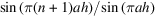is also called the geometric factor of the structure amplitude.

This size broadening is often modelled in practice by using an equation due to Scherrer. We now reproduce the simple derivation of the Scherrer equation following Klug & Alexander (1974).

Fig. 1.1.21shows the path-length difference versus the depth of the lattice plane. When the angle between the incoming beam and the lattice plane θ is different by an amountfrom the Bragg condition, it is always possible to find a lattice plane inside an infinite crystal where the extra path is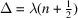for n integer, producing destructive interference. For a thick crystal this is true for arbitrarily small, which explains the sharp Bragg reflections. In the case of a crystal with finite dimensions, for smallthe plane for whichholds will not be reached, thus leading to an intensity distribution over some small angular range. We can use this idea to estimate the broadening of a Bragg reflection due to size effects.Figure 1.1.21 | top | pdf |Path-length difference of the scattered ray versus the depth of the lattice plane in the crystal. [Reproduced from Dinnebier & Billinge (2008) with permission from the Royal Society of Chemistry.]

The thickness of a crystallite in the direction perpendicular to p planes of separation dhkl (Fig. 1.1.21) is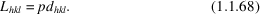The additional beam path between consecutive lattice planes at the angle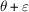is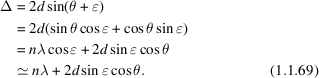The corresponding phase difference is then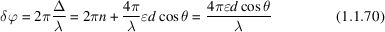and the phase difference between the top and the bottom layer (layer p) is then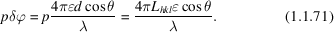Rearranging equation (1.1.71) leads to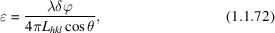which gives an expression for the misalignment angle in terms of the crystallite size Lhkl and the phase difference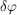between the reflections originating from the top plane and the bottom plane. Clearly, the scattered intensity is at a maximum for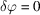(). With increasingthe intensity decreases, giving rise to a peak of finite width. Perfect cancellation of the waves from the top and bottom planes occurs for a phase difference of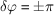, at which point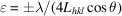. On a 2θ scale, the measured angular width between these points is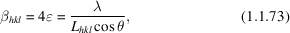giving us some measure of the peak width in radians that results from the finite particle size. A full treatment taking into account the correct form for the intensity distribution gives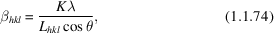with a scale factor of K = 0.89 for perfect spheres. In general K depends on the shape of the grains (e.g. K is 0.94 for cube-shaped grains), but it is always close to unity. This equation is not valid for crystallites3 that are too large or too small. In the case of large crystallites the peak width is governed by the coherence of the incident beam and not by particle size. For nanometre-sized crystallites, Bragg's law fails and the Debye equation needs to be used instead. The Debye equation (see Section 1.1.5.3) gives the scattering from an isotropically scattering sample such as a glass, liquid or powder, and does not presume that the sample is periodic.

1.1.4.1.2. Microstrain

| top | pdf |

Several important relationships in crystallography, including the effect of strain and microstrain on Bragg peaks, follow directly from a derivative of the Bragg equation (1.1.3). First we rewrite Bragg's law making the d-spacing the subject of the equation: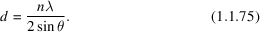The uncertainty of the measured lattice spacing is given by the total derivative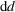,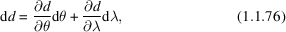leading to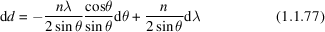and finally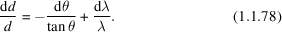When a crystal is strained, the d-spacings vary. A macroscopic strain changes the interplanar spacing by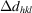, giving rise to a shift of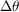in the average position of the diffraction peak. On the other hand, microscopic strains result in a distribution of d-spacings of width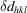, which has the effect of broadening the diffraction peak by. Equation (1.1.78) gives an expression for the amount of Bragg-peak broadening that occurs for a given.

References

Cheary, R. W. & Coelho, A. (1992). A fundamental parameters approach to X-ray line-profile fitting. J. Appl. Cryst. 25, 109–121.Google Scholar
Klug, H. P. & Alexander, L. E. (1974). X-ray Diffraction Procedures, 2nd ed. New York: John Wiley.Google Scholar